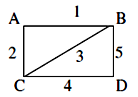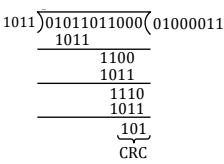# GATE Solved Paper 2017-19 - GATE 2017 Shift 1

>>>>>>>>GATE 2017 Shift 1

• Option : A
• Explanation :
A TM is recursive iff it halts for every input string (either in accept or reject state). Here, a computable function is defined in a similar way.

• A

(I) only• B

(II) only• C

Both (I) and (II)• D

Neither (I) nor (II)• Option : A
• Explanation :Shortest path from B to C are two B-A-C and B-C both of weight '3'

• A

x = 1, y = 2• B

x = 2, y = 1• C

x = 2, y = 2• D

x = 1, y = 1• Option : D
• Explanation : Reentrant property is provided, so that a process who owns a lock, can acquire same lock multiple times. Here it is non-reentrant as given, process cant own same lock multiple times. So if a thread tries to acquire already owned lock, will get blocked, and this is a deadlock.
Hence correct answer is x=1, y=1.

• A

01011011010• B

01011011011• C

01011011101• D

01011011100• Option : C
• Explanation :• A

a tautology• B

a contradiction• C

always TRUE when p is FALSE• D

always TRUE when q is TRUE• Option : D
• Explanation : (p → q) → r is contradiction only when  P q r T T F F T F F F F
And now for the above combination, the expression is always true when q is true. When q is false in the above combination (third one) will be false.
Related Quiz.
GATE 2017 Shift 1